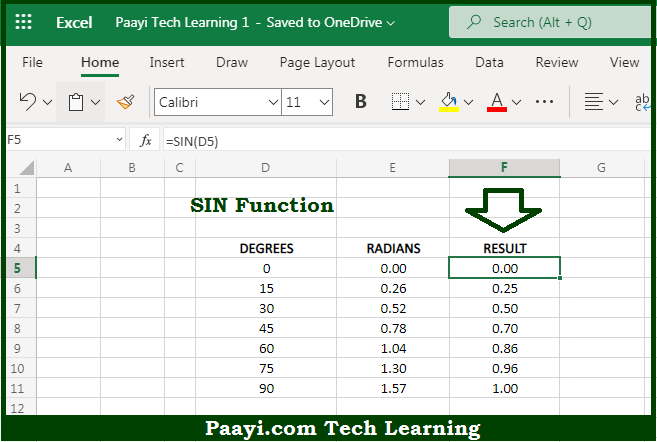# Learn How to Use Microsoft Excel SIN Function

Written by | 0 Comments | 813 Views

In this article, you will learn how to use the Microsoft Excel SIN function and its prime function in Microsoft Excel. You will also get to know the Microsoft Excel SIN function return value and syntax with the help of some examples.

Microsoft Excel SIN Function

The main purpose of the Microsoft Excel SIN function is to get the sine of the angle provided in radians. That implies, with the help of the SIN function you can able to return the sine of an angle provided in radians. In geometry, the sine of an angle returns the ratio of a right triangle's opposite side over its hypotenuse. You need to multiply the angle by PI()/180 or use the RADIANS function to convert to radians, to provide an angle to SIN in degrees. So, with the help of the SIN function, you can able to get the sine of an angle provided in the radians.

Return Value of SIN Function

The return value will be the sine value.

Syntax of SIN Function

=SIN(number)

Where the arguments:

• number: This is the angle in radians of which you want to get the sine.

How to Use Microsoft Excel SIN Function?So we know that Microsoft Excel SIN function you can able to get the sine of the angle. That implies, with the help of the SIN function you can able to return the sine of an angle provided in radians. In geometry, the sine of a right triangle's angle is equal to the ratio of the length of its hypotenuse over the length of its adjacent side. You need to multiply the angle by PI()/180 or use the RADIANS function to convert to radians, to provide an angle to SIN in degrees. So, with the help of the SIN function, you can able to get the sine of an angle provided in the radians.